#### Option 3.2.10. Histogram

Select Histogram in the Calculation field on the Virtual tab.

A Histogram point records the frequency at which the value of the source point, identified in the Expression field, updates within specified range intervals. This information is typically displayed graphically as a histogram.

Each time the source point is updated, the counter whose range encompasses the source point value is incremented.

You must configure a Histogram point as an array point. The number of elements in the array will be:

the number of range intervals you desire + 6

The extra six elements are used to maintain the: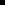Minimum of the point values receivedMaximum of the point values receivedTotal number of samples receivedSum of the values of all samples receivedNumber of sample values that were less than the lower limit of the point range (underflow bucket)Number of sample values that were greater than the upper limit of the point range (overflow bucket)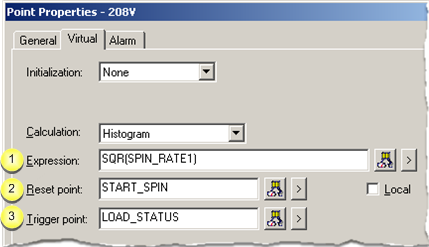Expression Reset point Trigger point Example

 Expression

Enter an expression that calculates the value of the Histogram point.

The expression will be monitored for transitions.

The expression may contain:One or more Point IDs as well asConstant values, operations, and functions.

Buttons to help with the equation are as follows.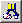Opens the Expression Editor. Displays a Popup menu to: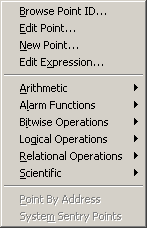Open: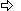The Select a Point browser.The Point Properties dialog box for a selected point.A New Point dialog box.The Expression Editor.Display an extended menu with the associated operations.

 Reset point

Based on whether there is an entry in the Reset Point field, at runtime the Histogram point is reset as follows:

 When the trigger point is not defined If And Then An Initial Histogram Value is The Current value of the Expression is Each Array element of the Histogram is reset to: Not defined Unavailable Zero Not defined Available Zero Defined Unavailable Initial value Defined Available Initial value When the trigger point is defined If Then An Initial Histogram Value is Each Array element of the Histogram is reset to: Not defined Zero Defined Initial value

Note: Buttons to the right of the Reset Point and Trigger Point fields help with configuration.Opens the Select a Point browser to select an available point. Displays a Popup menu to: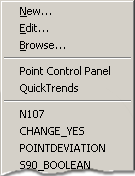Open:A New Point dialog box.The Point Properties dialog box for a selected point.The Select a Point browser.Select a recently selected point.

 Trigger point

When the value of the Histogram point updates depends on whether or not you defined the trigger point as follows:

 Trigger point The Value of the Histogram is Updated When the Value of the: Defined Trigger point is updated. Not defined Source point in the Expression field is updated.

Buttons to help with the trigger point have the same functionality as the buttons to the right of the Reset Point field.

 Histogram Point Example

A point called CYCLE_TIME is expected to have a value between 50 and 100, and you want to track the frequency at which values occur within five intervals.

Create a Histogram point called HIST_CYCLE_TIME with:

 Display Low 50 Display High 100 Elements 11 Expression CYCLE_TIME

The eleven elements of this point contain the following information, where X is the number of point value samples:

 Element Contents HIST_CYCLE_TIME X<50 (underflow bucket) HIST_CYCLE_TIME 60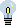Tip: Use the Display Limits fields to specify the lower and upper range values within which the point values are expected to occur. The range intervals are automatically calculated based on the Display High and Display Low limits and the number of Elements you specify.

 Step 3.2. Configure virtual calculations.